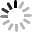NEWS
Home > news > Company news about What controls the pulse frequency of the stepping motor? How to control the pulse frequency?
EVENTS
86-020-36907671
Contact Now

# What controls the pulse frequency of the stepping motor? How to control the pulse frequency?

2023-01-06Due to its unique structure, the stepper motor is marked with "inherent step angle of the motor" when leaving the factory (for example, 0.9 °/1.8 °, which means that the angle of each step of half step operation is 0.9 °, and 1.8 ° for full step operation).

However, in many precision control and occasions, the angle of the whole step is too large, which affects the control accuracy, and the vibration is too large. Therefore, it is required to complete the inherent step angle of the motor in many steps, which is called subdivision drive. The electronic device that can achieve this function is called subdivision drive.

V=P* θ e÷360*m
5: Motor speed (r/s) P: pulse frequency (Hz) θ e: Inherent step angle of motor m: subdivision (full step is 1, half step is 2)

The rotation angle of the stepping motor is calculated independent of the signal frequency. The number of pulses is 10. The step angle of the stepping motor is 1.8 degrees. Then the stepping motor should rotate 18 degrees.

Pulse refers to a cycle of motor coil level from high to low, or from low to high. A few conversion cycles are several pulses, and the frequency is the number of conversions in a second, not the number of energizations in a second. If the frequency of the pulse signal sent by the plc is 50HZ, it means that the speed of the stepping motor to execute the number of pulses is 50 cycles within one second.

The pulse signal is the electric reading source of the stepping motor, which is characterized by discontinuity. Every time the stepping motor receives a pulse signal, it rotates at a certain angle. The controller sends a certain number of pulse signals, and the motor rotates at a certain angle. The high pulse frequency makes the motor rotate quickly. One is the total quantity, and the other is the quantity per second, which is the difference.

Send your inquiry directly to us